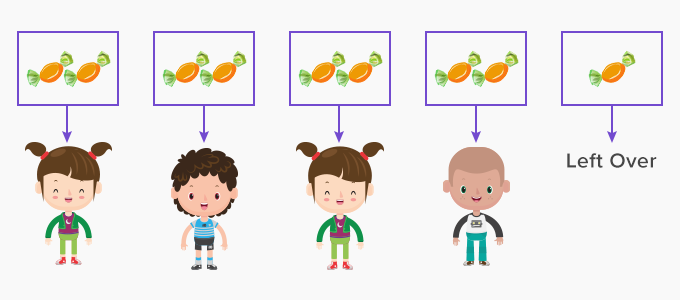# Remainder - Definition with Examples

The Complete K-5 Math Learning Program Built for Your Child

• 30 Million Kids

Loved by kids and parent worldwide

• 50,000 Schools

Trusted by teachers across schools

• Comprehensive Curriculum

Aligned to Common Core

What is Remainder?

Remainder means something which is ‘left over’ or ‘remaining’.

If you have 9 toffees and you share it equally with your four friends. How many toffees will you have?If you give two toffees each to your friends, you would have shared 8 toffees.  Only1 toffee will remain with you, and this leftover of 1 toffee is called the remainder.Mathematically we can write the above expression as:

9 ÷ 4 = 2 remainder 1

Where 9 is the dividend, 4 is the divisor, 2 is the quotient, and 1 is the remainder.

On dividing 22 by 3.

We get 3 equal parts of 7, that add up to 21

3 x 7 = 21.

We are left with 1. This 1 is the remainder.

When one number cannot divide another number completely, it le get a remainder.

Examples:

 18 ÷ 7 Remainder 4 15 ÷ 10 Remainder 5 23 ÷ 6 Remainder 5 46 ÷ 9 Remainder 1 15 ÷ 5 Remainder 0

Properties of Remainder:

• When one number divides another number completely, the remainder is 0.

• The remainder is always less than the divisor. If the remainder is greater than the divisor, it means that the division is incomplete.

• It can be greater than or lesser than the quotient. For example; when 41 is divided by 7, the quotient is 5 and the remainder is 6. Here the remainder is greater than the quotient.

 Fun Facts The word remainder comes from the French word ‘remaindre’ which means to remain.

Won Numerous Awards & Honors